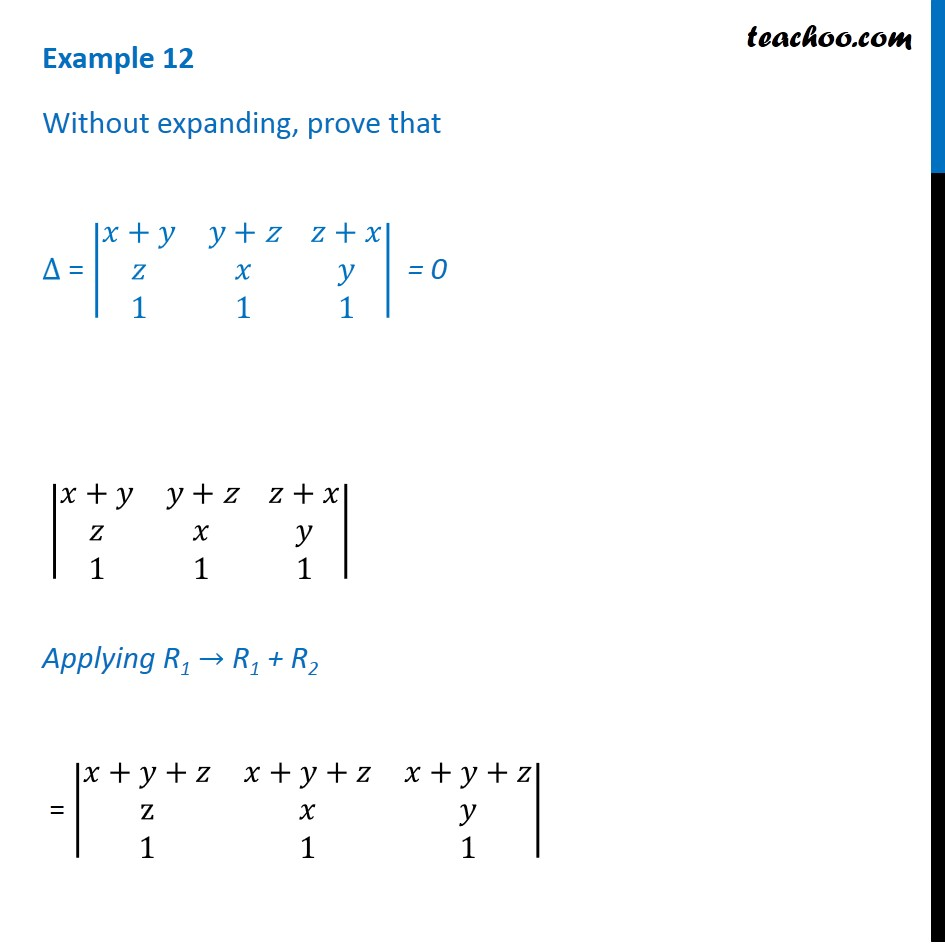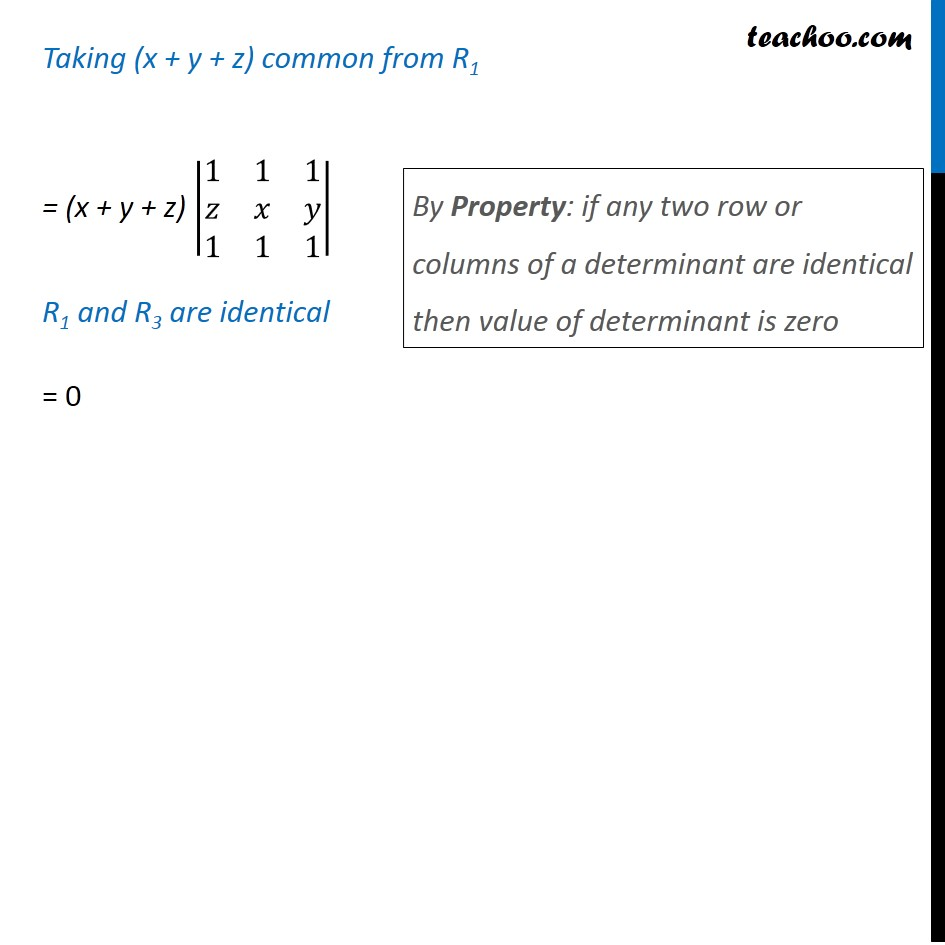1. Chapter 4 Class 12 Determinants (Term 1)
2. Serial order wise
3. Examples

Transcript

Example 12 Without expanding, prove that ∆ = |■8(𝑥+𝑦&𝑦" + z" &𝑧+𝑥@𝑧&𝑥&𝑦@1&1&1)| = 0 |■8(𝑥+𝑦&𝑦" + z" &𝑧+𝑥@𝑧&𝑥&𝑦@1&1&1)| Applying R1 → R1 + R2 = |■8(𝑥+𝑦+𝑧&𝑥+𝑦+𝑧&𝑥+𝑦+𝑧@z&𝑥&𝑦@1&1&1)| Taking (x + y + z) common from R1 = (x + y + z) |■8(1&1&1@𝑧&𝑥&𝑦@1&1&1)| R1 and R3 are identical = 0 By Property: if any two row or columns of a determinant are identical then value of determinant is zero

Examples